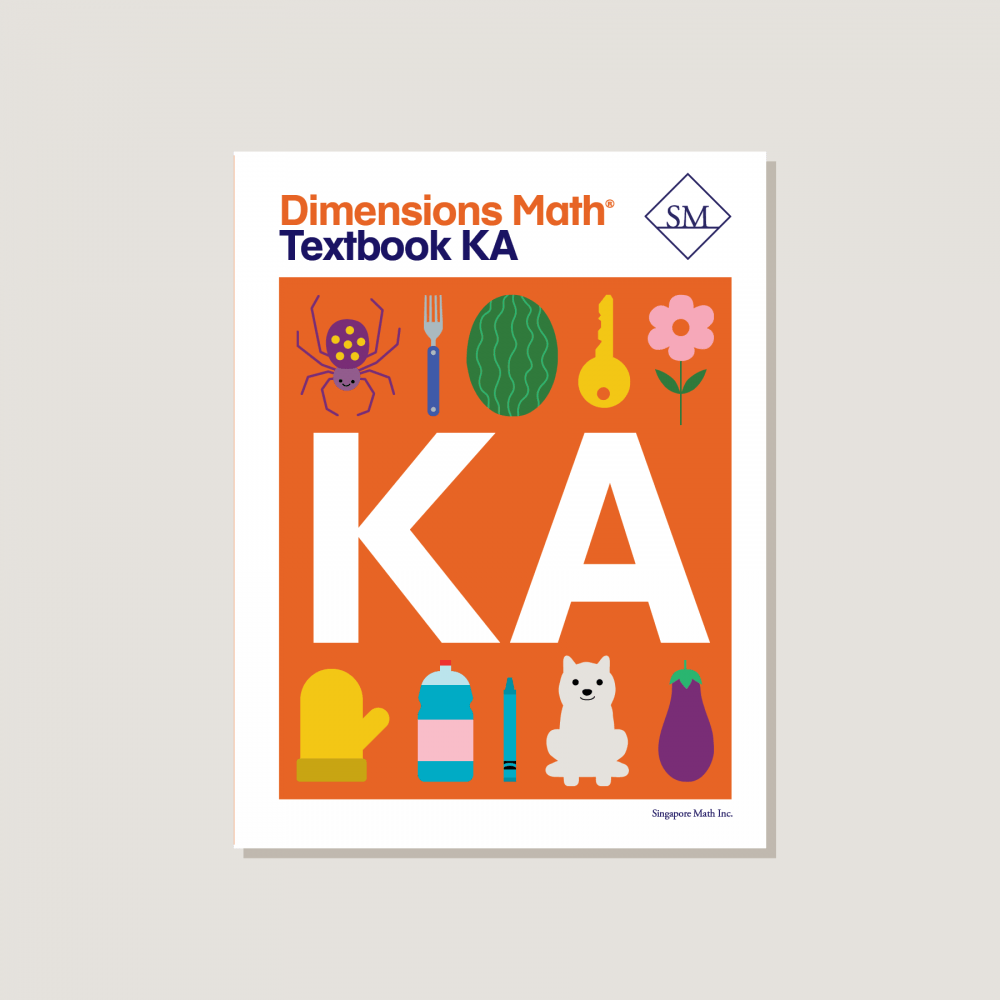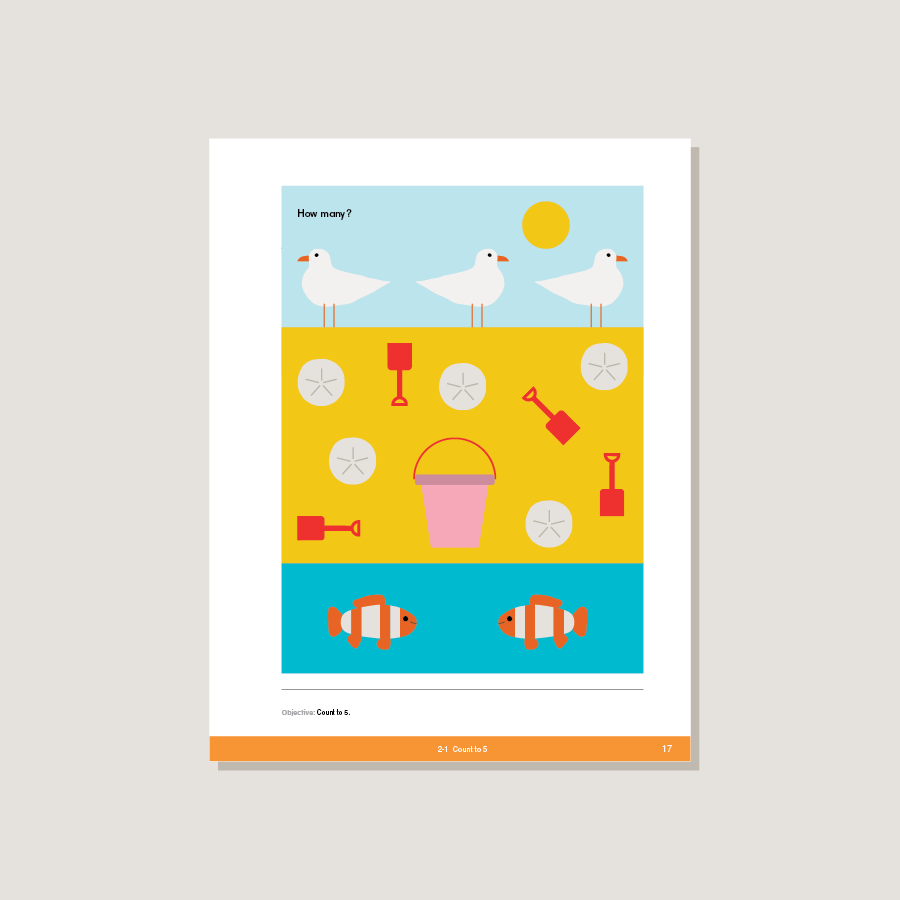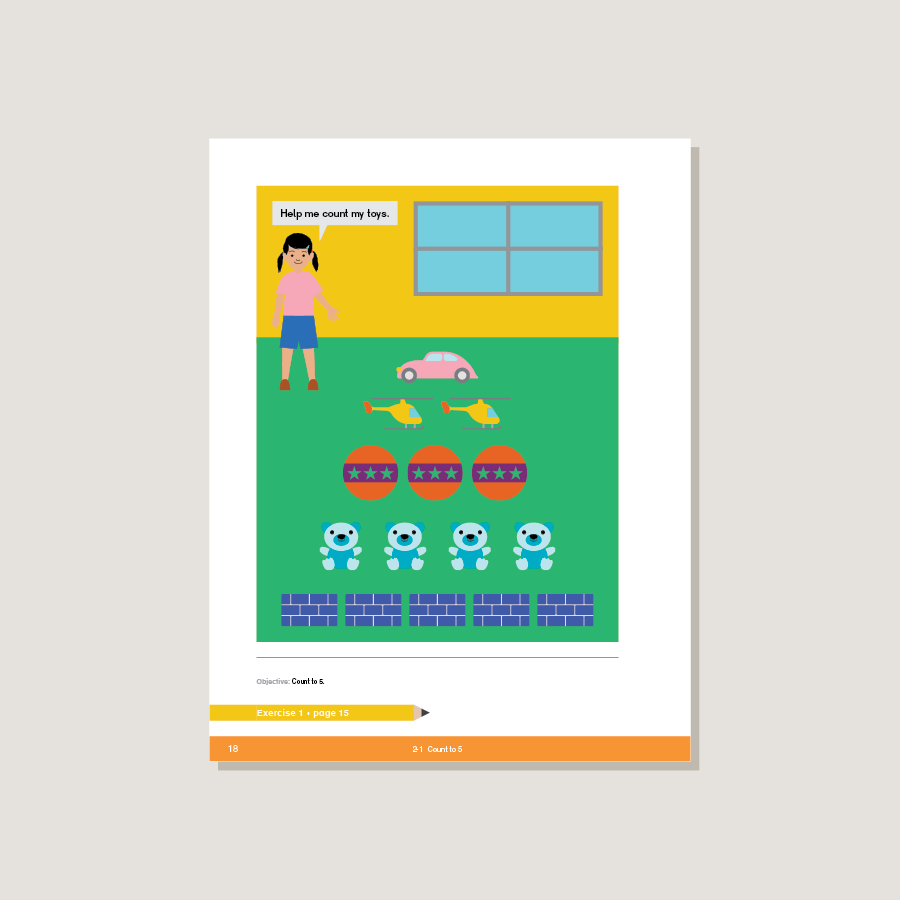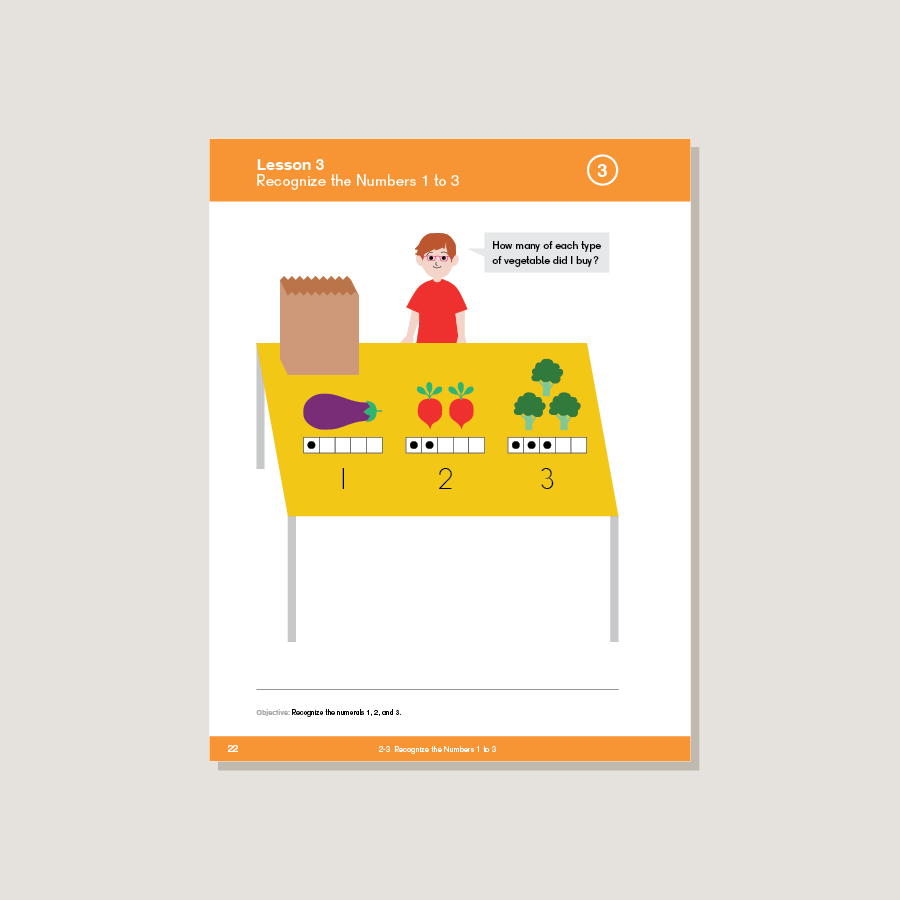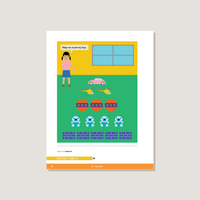# Dimensions Math Textbook KA

\$13.70

Textbooks develop concepts in increasing levels of abstraction. Each topic is thoughtfully introduced and extended through the use of exploration, play, and opportunities for mastery of skills. Features & Components:
• Chapter Opener: Each chapter begins with an engaging scenario that stimulates student curiosity in new concepts. This scenario also provides teachers an opportunity to review skills.
• Lesson: Engaging imagery draws students into the concept of each lesson.
• Exercise: A pencil icon at the end of the lesson links to additional practice problems in the corresponding workbook.
• Review: A review of chapter material provides ongoing practice of concepts and skills.
Note: Two textbooks (A and B) for each grade correspond to the two halves of the school year. Answer key not included. Soft cover.
SKU: DMTKA

ISBN: 9781947226029

Pagecount: 152

Dimensions: 8.5 x 11 x 0.75 in

Binding: Standard

Color: Color

Cover: Soft

Perforated: No

Sample Pages

Chapter 1: Match, Sort, and Classify
Lesson 1: Left and Right
Lesson 2: Same and Similar
Lesson 3: Look for One That is Different
Lesson 4: How Does it Feel?
Lesson 5: Match the Things That Go Together
Lesson 6: Sort
Lesson 7: Practice

Chapter 2: Numbers to 5
Lesson 1: Count to 5
Lesson 2: Count Things up to 5
Lesson 3: Recognize the Numbers 1 to 3
Lesson 4: Recognize the Numbers 4 and 5
Lesson 5: Count and Match
Lesson 6: Write the Numbers 1 and 2
Lesson 7: Write the Number 3
Lesson 8: Write the Number 4
Lesson 9: Trace and Write 1 to 5
Lesson 10: Zero
Lesson 11: Picture Graphs
Lesson 12: Practice

Chapter 3: Numbers to 10
Lesson 1: Count 1 to 10
Lesson 2: Count Up to 7 Things
Lesson 3: Count Up to 9 Things
Lesson 4: Count Up to 10 Things — Part 1
Lesson 5: Count Up to 10 Things — Part 2
Lesson 6: Recognize the Numbers 6 to 10
Lesson 7: Write the Numbers 6 and 7
Lesson 8: Write the Numbers 8, 9, and 10
Lesson 9: Write the Numbers 6 to 10
Lesson 10: Count and Write the Numbers 1 to 10
Lesson 11: Ordinal Positions
Lesson 12: One More Than
Lesson 13: Practice

Chapter 4: Shapes and Solids
Lesson 1: Curved or Flat
Lesson 2: Solid Shapes
Lesson 3: Closed Shapes
Lesson 4: Rectangles
Lesson 5: Squares
Lesson 6: Circles and Triangles
Lesson 7: Where is It?
Lesson 8: Hexagons
Lesson 9: Sizes and Shapes
Lesson 10: Combine Shapes
Lesson 11: Graphs
Lesson 12: Practice

Chapter 5: Compare Height, Length, Weight, and Capacity
Lesson 1: Comparing Height
Lesson 2: Comparing Length
Lesson 3: Height and Length — Part 1
Lesson 4: Height and Length — Part 2
Lesson 5: Weight — Part 1
Lesson 6: Weight — Part 2
Lesson 7: Weight — Part 3
Lesson 8: Capacity — Part 1
Lesson 9: Capacity — Part 2
Lesson 10: Practice

Chapter 6: Comparing Numbers Within 10
Lesson 1: Same and More
Lesson 2: More and Fewer
Lesson 3: More and Less
Lesson 4: Practice — Part 1
Lesson 5: Practice — Part 2

A & B Books: Our programs divide the school year into two semesters. “A” level books are for the first half of the school year. “B” level books are for the second half of the school year. You need both “A” and “B” material for a complete school year.

Required Components: Textbooks, Workbooks, and Guides (either Home Instructor’s Guides or Teacher’s Guides) are all necessary components. These three elements each serve a unique function and work together to build math mastery.

Printouts: Dimensions Math PK-5 Resources

Recommended Manipulatives: Dimensions Math Grades PK-5 Recommended Manipulatives

Answer Key: Located in corresponding Teacher’s Guide

Dimensions Math At Home™ Videos

Invite a professional Singapore math teacher into your home classroom. This subscription of pre-recorded lessons covers all Textbook and Workbook material for an entire school year and can be done at your own pace.

Manipulatives

Manipulatives help students visualize and represent math concepts. Stock up on suggested items to deepen engagement during math activities and lessons.

Supplementary Math

Supplementary math helps students of all abilities: struggling, advanced, or on level. Find just the right addition to round out your core program.